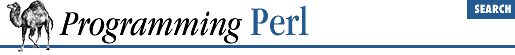home | O'Reilly's CD bookshelfs | FreeBSD | Linux | Cisco | Cisco Exam

#### 3.2.131 scalar

```scalar ```

EXPR
```
```

This pseudo-function may be used within a ``` LIST ``` to force ``` EXPR ``` to be evaluated in scalar context when evaluation in list context would produce a different result.

For example:

`local(\$nextvar) = scalar <STDIN>;`

prevents ``` <STDIN>``` from reading all the lines from standard input before doing the assignment, since assignment to a local list provides a list context. (Without the use of scalar in this example, the first line from ``` <STDIN>``` would still be assigned to ``` \$nextvar``` , but the subsequent lines would be read and thrown away. This is because the assignment is being made to a list - one that happens to be able to receive only a single, scalar value.)

Of course, a simpler way with less typing would be to simply leave the parentheses off, thereby changing the list context to a scalar one:

`local \$nextvar = <STDIN>;`

Since a print function is a ``` LIST ``` operator, you have to say:

`print "Length is ", scalar(@ARRAY), "\n";`

if you want the length of ``` @ARRAY``` to be printed out.

One never needs to force evaluation in a list context, because any operation that wants a list already provides a list context to its list arguments for free. So there's no list function corresponding to scalar .